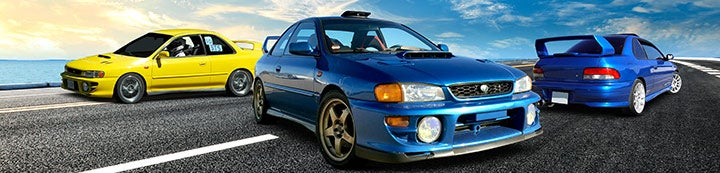1 - 3 of 3 Posts

#### clsmooth

·
##### Registered
00 BRP RS Coupe
Joined
·
663 Posts
Discussion Starter
Has anyone calculated what the volume is of the spare tire compartment (with the spare tire removed)?

#### winged1

·
##### Registered
2000 Subaru Impreza 2.5RS
Joined
·
12 Posts
No, but the formula is pretty simple. It's just a cylinder. To calc in cubic inches:

I pasted a more complete step by step that I got from https://www.hunker.com/13401375/how-to-calculate-concrete-needed-for-a-cylinder

Step 1
Measure the diameter of the cylinder. To measure the diameter, measure one circular end of the cylinder across the widest point. Your ruler or measuring tape should cross the center of the circle. Record the measurement in inches.

Step 2
Use your calculator to divide the length of diameter by 2. The result is the length of the radius. For example, if the measured diameter is 12 inches, the radius is 6 inches. Record the length of the radius.

Step 3
Measure the height of the cylinder in inches and record the result.

Step 4
Multiply the length of the radius by itself. For example, if your radius measures 6 inches, multiply 6 by 6, for a total of 36. Record the result of this calculation.

Step 5
Use the number 3.142 for the value of pi. Multiply the result of step 4 by 3.142 and record the result. For example, with a radius of 6, the result is 113.112.

Step 6
Multiply the result of step 5 by the height of the cylinder. This calculation will provide the volume of the cylinder in cubic inches. For example, if a cylinder 12 inches in diameter has a height of 40 inches, the result is a volume of 4523.48 cubic inches.

#### clsmooth

·
##### Registered
00 BRP RS Coupe
Joined
·
663 Posts
Discussion Starter